ISEE Lower Level Math : How to find the area of a square

Example Questions

1 2 3 5 Next →

Example Question #1191 : Isee Lower Level (Grades 5 6) Mathematics Achievement

Find the area of a square that has a base of length 6 feet.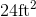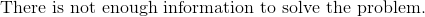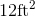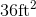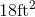Explanation:

To find the area of a square, we will use the following formula: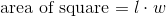where l is the length and w is the width of the square.

Now, we know the square has a base of length 6 feet.  Because it is a square, we know that all sides are equal.  Therefore, all sides are 6 feet.

Knowing this, we can substitute into the formula.  We get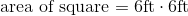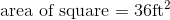Example Question #1197 : Isee Lower Level (Grades 5 6) Mathematics Achievement

Use the following square to answer the question: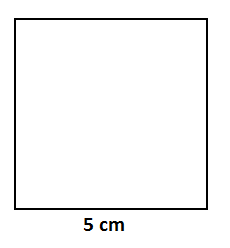Find the area.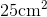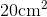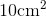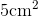Explanation:

To find the area of a square, we will use the following formula:where l is the length and w is the width of the square.

Now, let's look at the square.We can see the length is 5cm.  Because it is a square, all sides are equal.  Therefore, the width is also 5cm.

Knowing this, we can substitute into the formula.  We get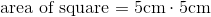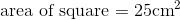Example Question #1198 : Isee Lower Level (Grades 5 6) Mathematics Achievement

Find the area of a square with a width of 7in.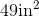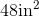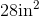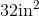Explanation:

To find the area of a square, we will use the following formula:where l is the length and w is the width of the square.

Now, we know the width of the square is 7in.  Because it is a square, we know that all sides are equal.  Therefore, the length is also 7in.

Knowing this, we can substitute into the formula.  We get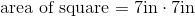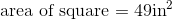Example Question #1199 : Isee Lower Level (Grades 5 6) Mathematics Achievement

Use the following square to answer the question: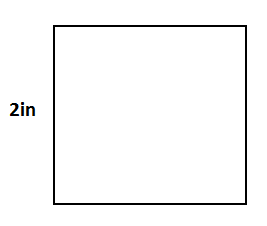Find the area.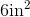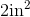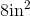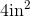Explanation:

To find the area of a square, we will use the following formula:where l is the length and w is the width of the square.

Now, given the squareWe can see the width is 2in.  Because it is a square, all sides are equal.  Therefore, the length is also 2in.

Knowing this, we can substitute into the formula.  We get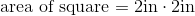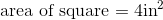Example Question #261 : Geometry

Find the area of a square that has a width of 10cm.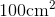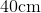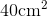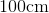Explanation:

To find the area of a square, we will use the following formula:where l is the length and w is the width of the square.

Now, we know the width of the square is 10cm.  Because it is a square, we know that all sides are equal.  Therefore, the length is also 10cm.

Knowing this, we will substitute into the formula.  We get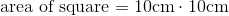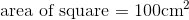Example Question #41 : How To Find The Area Of A Square

Find the area of a square with a length of 13in.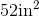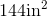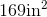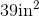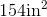Explanation:

To find the area of a square, we will use the following formula:where l is the length and w is the width of the square.

Now, we know the length of the square is 13in.  Because it is a square, all sides are equal.  Therefore, the length is also 13in.

Knowing this, we can substitute into the formula.  We get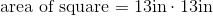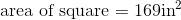Example Question #111 : Squares

Find the area of a square with a length of 11in.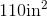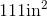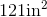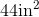Explanation:

To find the area of a square, we will use the following formula: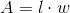where l is the length and w is the width of the square.

Now, we know the length of the square is 11in.  Because it is a square, all sides are equal.  Therefore, the width is also 11in.

Knowing this, we can substitute into the formula.  We get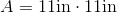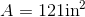Example Question #43 : How To Find The Area Of A Square

Find the length of one side of a square with an area of 64in.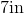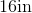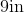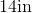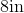Explanation:

The formula to find area of a square is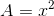where x is the length of one side of a square.  Now, to find the length of a side, we will solve for x.

So, we know the area of the square is 64in.  Knowing this, we can substitute into the formula.  We get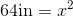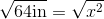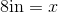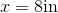Therefore, the length of one side of the square is 8in.

Find the area of a square with a width of 7cm.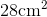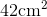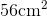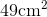Explanation:

To find the area of a square, we will use the following formula:where l is the length and w is the width of the square.

Now, we know the width of the square is 7cm.  Because it is a square, all sides are equal.  Therefore, the length is also 7cm.  So, we get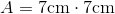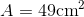1 2 3 5 Next →